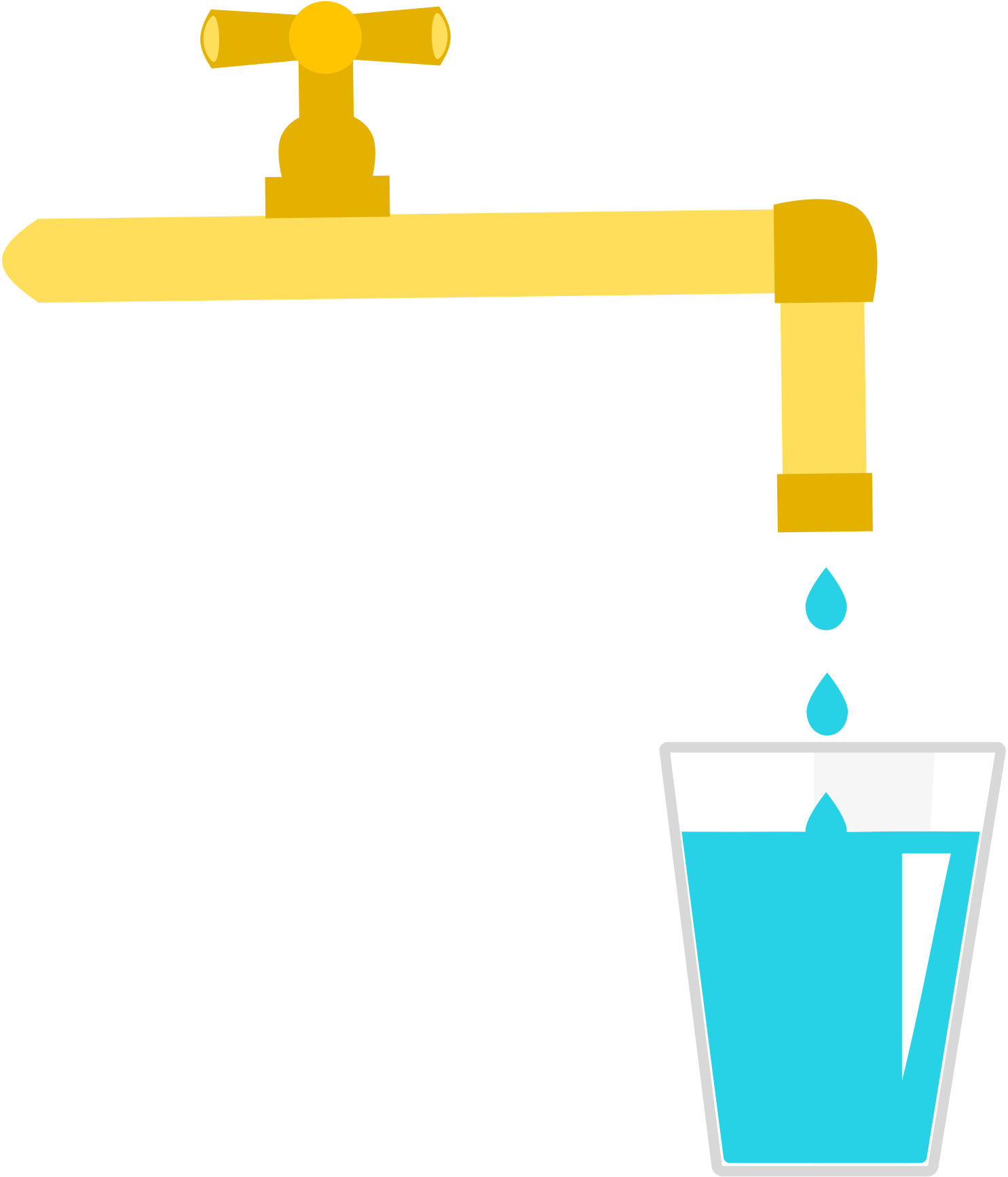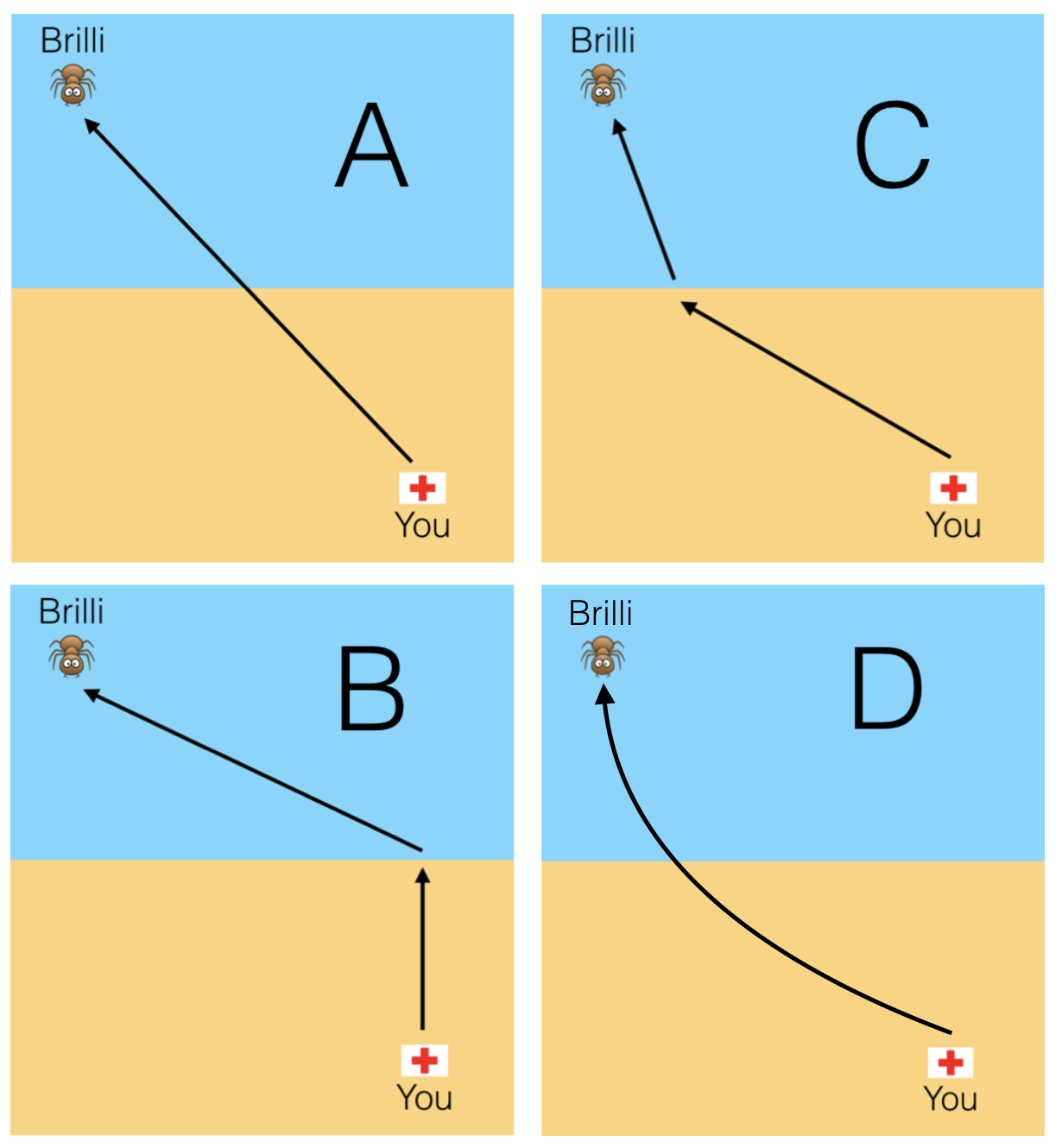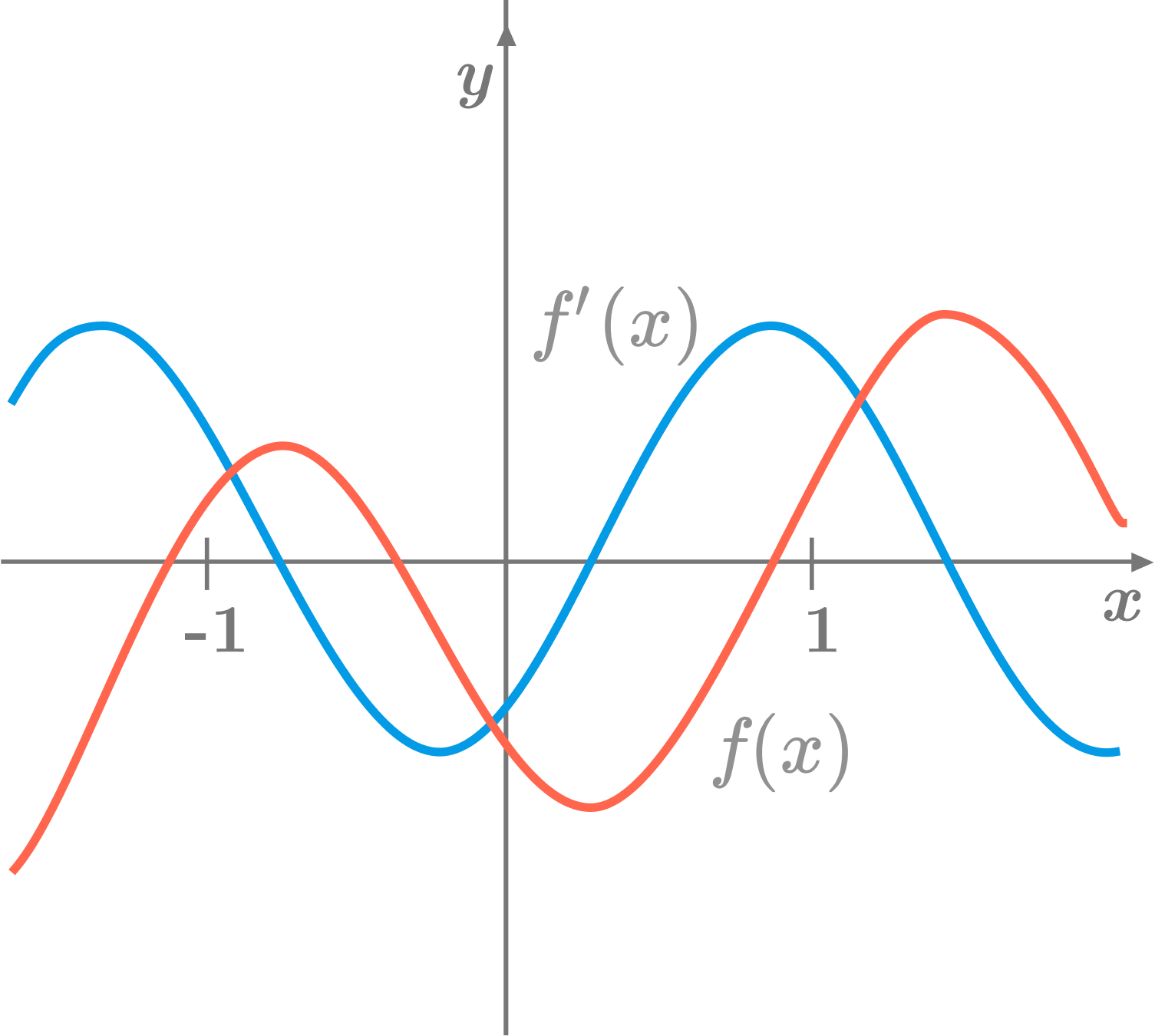Calculus

# Calculus Warmups: Level 2 ChallengesWater drips into the cup, whose shape is shown in the image, at a steady rate.

What can we say about the rate of change of the height of water level?

You have just started your summer job as a lifeguard at the beach, which has oversized ants leisurely swimming. You are on top of your lifeguard stand and notice that Brilli the ant is making distress signals some distance offshore, and you want to travel to Brilli in the quickest way possible.Knowing that you travel faster running over sand than you do swimming through water, which of the above best illustrates the quickest path to take?

Assume that the water is still, with no currents.In the above diagram, the red curve is the graph of a function $f,$ and the blue curve is the graph of its first derivative $f'.$ What is the relationship between $f'(-1)$ and $f''(1) ?$

What is the value of

$\int_{-1} ^ 1 \frac{1}{x} \, dx ?$

$1 + \frac {1}{\color{#3D99F6}2} + \frac {1}{\color{#3D99F6}6} + \frac {1}{\color{#3D99F6}{12}} + \frac {1}{\color{#3D99F6}{20}} + \ldots = \ \color{teal}?$

×